# CIE A Level Chemistry复习笔记4.1.4 Molecular Ion Peak & Fragmentation

### Mass Spectrometry: Deducing Molecular Formula

• Each peak in the mass spectrum corresponds to a certain fragment with a particular m/e value
• The peak with the highest m/e value is the molecular ion (M+) peak which gives information about the molecular mass of the compound
• The molecular ion is the entire molecule that has lost one electron when bombarded with a beam of electrons• The [M+1] peak is a smaller peak which is due to the natural abundance of the isotope carbon-13
• The height of the [M+1] peak for a particular ion depends on how many carbon atoms are present in that molecule; the more carbon atoms, the larger the [M+1] peak is
• For example, the height of the [M+1] peak for an hexane (containing six carbon atoms) ion will be greater than the height of the [M+1] peak of an ethane (containing two carbon atoms) ion

#### Worked example: Analysing mass spectra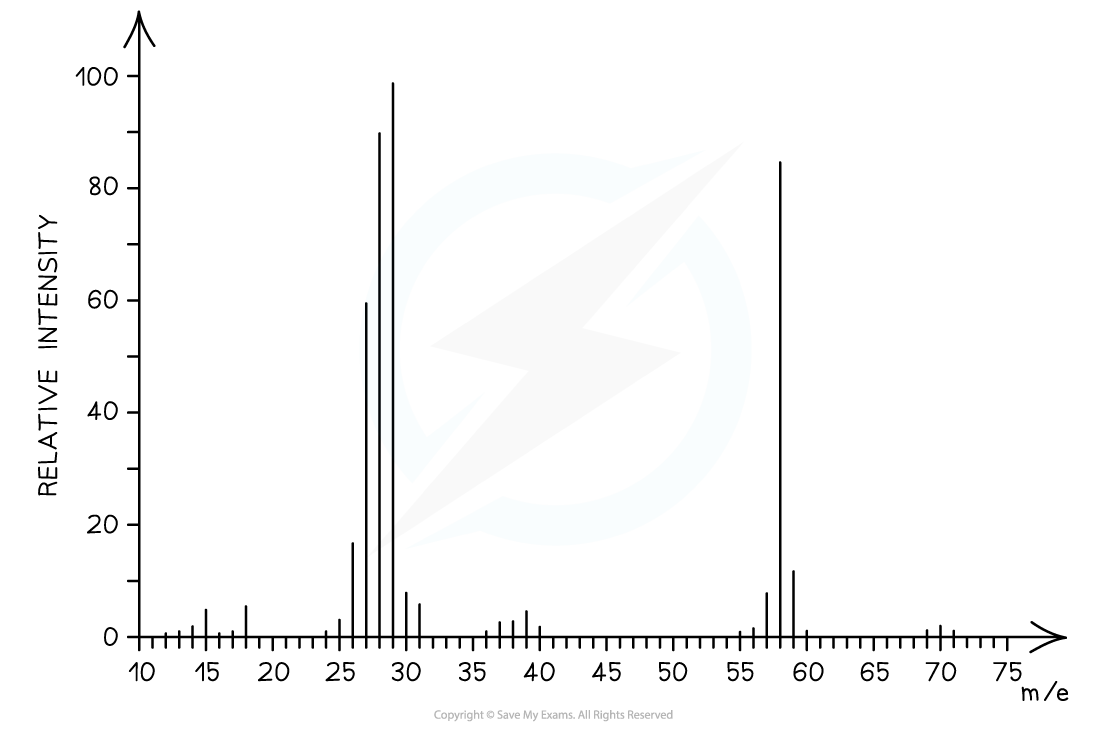The mass spectrum corresponds to propanal as the molecular ion peak is at m/e = 58

Propanal arises from the CH3CH2CHO+ ion which has a molecular mass of 58

Butanal arises from the CH3CH2CH2CHO+ ion which has a molecular mass of 72

### Identifying Molecules using Fragmentation

• The molecular ion peak can be used to identify the molecular mass of a compound
• However, different compounds may have the same molecular mass
• To further determine the structure of the unknown compound, fragmentation is used
• Fragments may appear due to the formation of characteristic fragments or the loss of small molecules
• For example, a peak at 29 is due to the characteristic fragment C2H5+­­
• Loss of small molecules give rise to peaks at 18 (H2O), 28 (CO), and 44 (CO2)

#### Alkanes

• Simple alkanes are fragmented in mass spectroscopy by breaking the C-C bonds
• M/e values of some of the common alkane fragments are given in the table below

m/e values of fragments table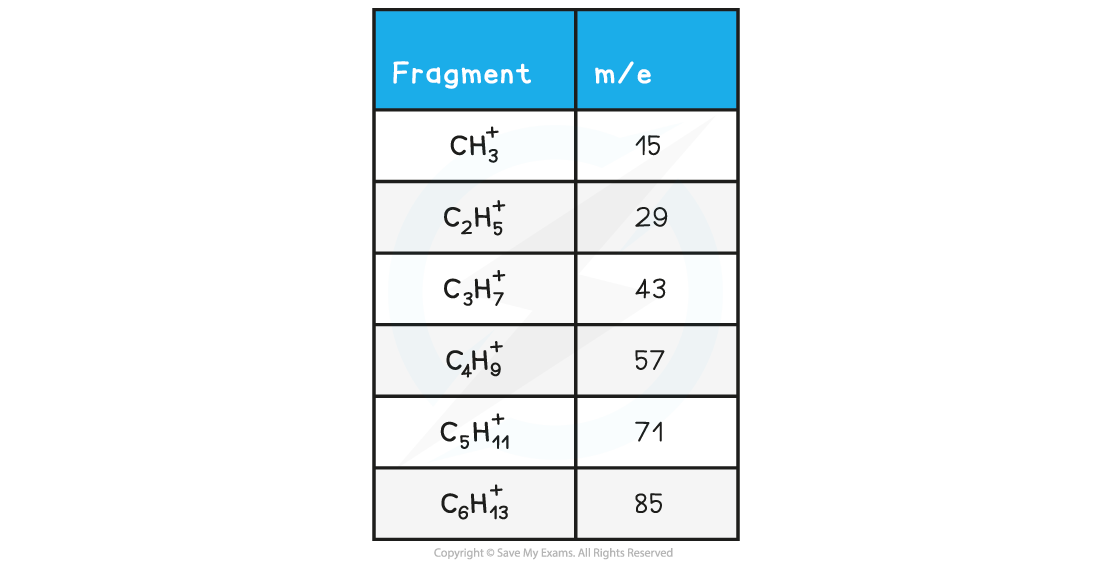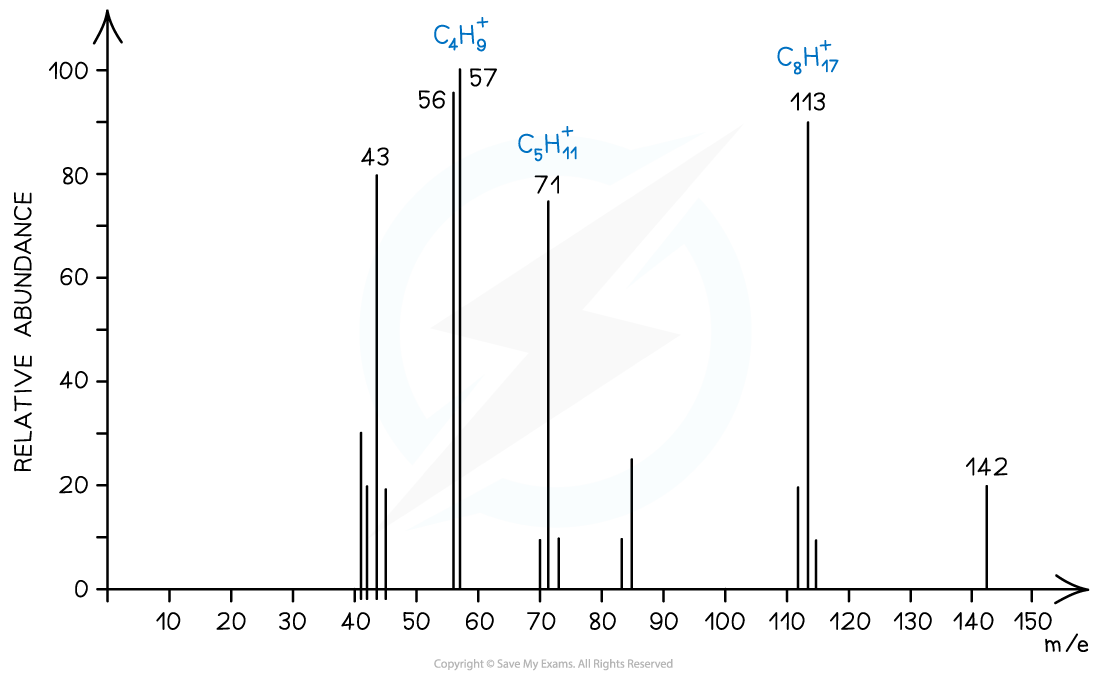Mass spectrum showing fragmentation of alkanes

#### Halogenoalkanes

• Halogenoalkanes often have multiple peaks around the molecular ion peak
• This is caused by the fact that there are different isotopes of the halogens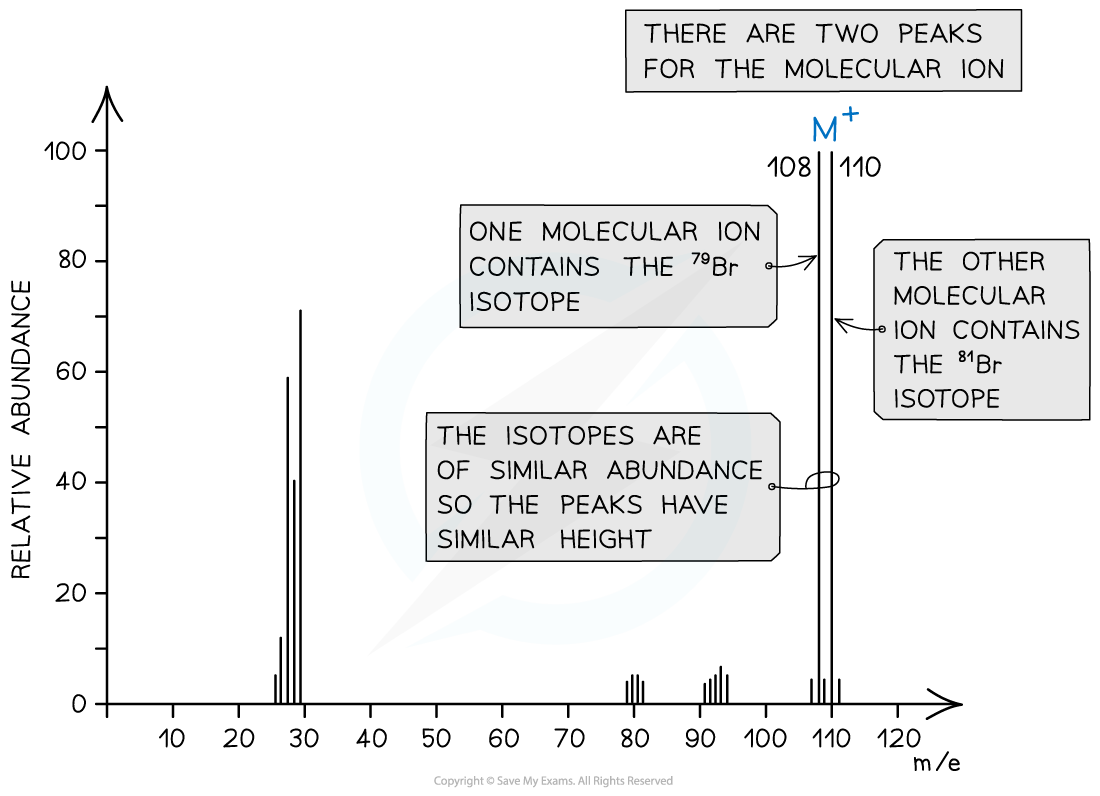Mass spectrum showing different isotopes of the halogens in the molecular ion

#### Alcohols

• Alcohols often tend to lose a water molecule giving rise to a peak at 18 below the molecular ion
• Another common peak is found at m/e value 31 which corresponds to the CH2OH+­­ fragment
• For example, the mass spectrum of propan-1-ol shows that the compound has fragmented in four different ways:
• Loss of H• to form a C3H7O+ fragment with m/e = 59
• Loss of a water molecule to form a C3H6+ fragment with m/e = 42
• Loss of a •C2H5 to form a CH2OH+ fragment with m/e = 31
• And the loss of •CH2OH to form a C2H5+ fragment with m/e = 29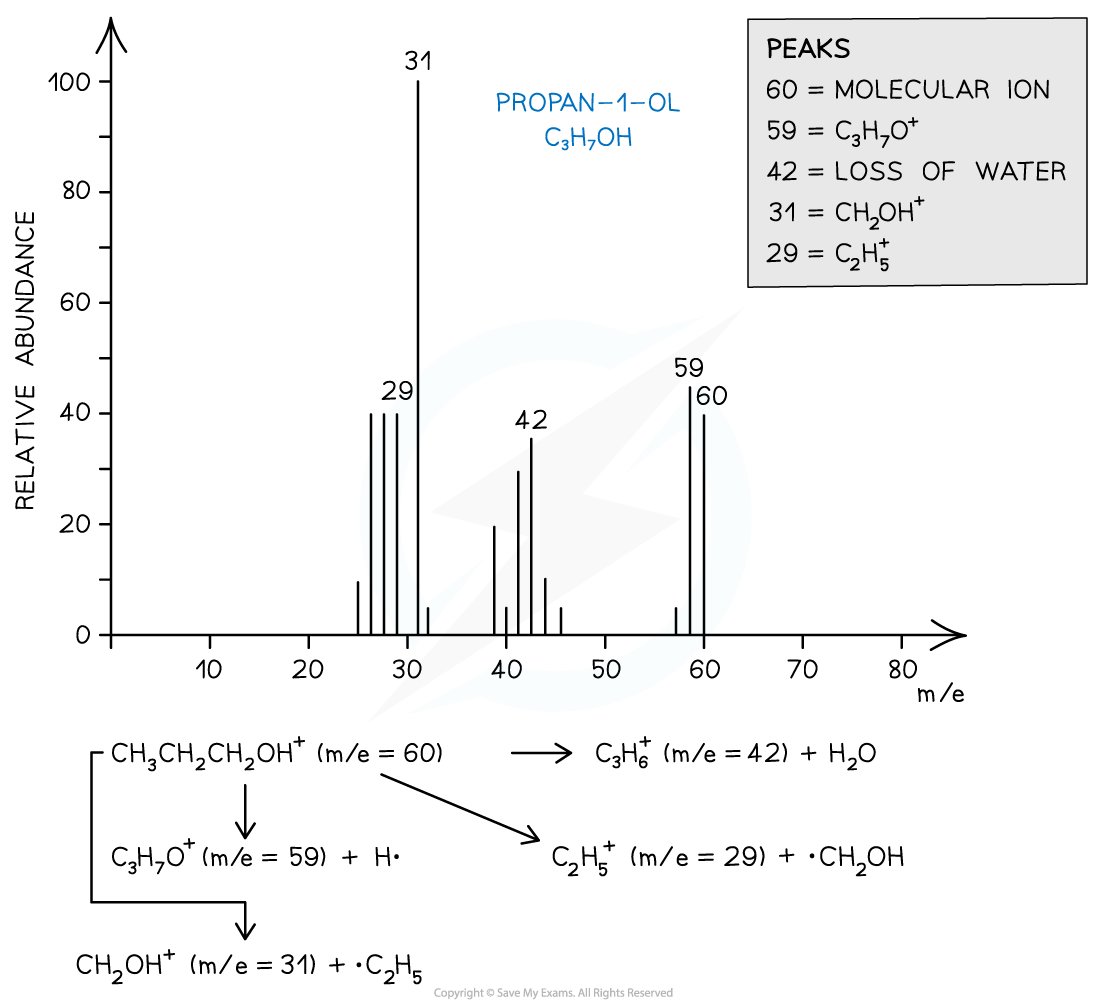Mass spectrum showing the fragmentation patterns in propan-1-ol (alcohol)

#### Worked example: Ion fragmentation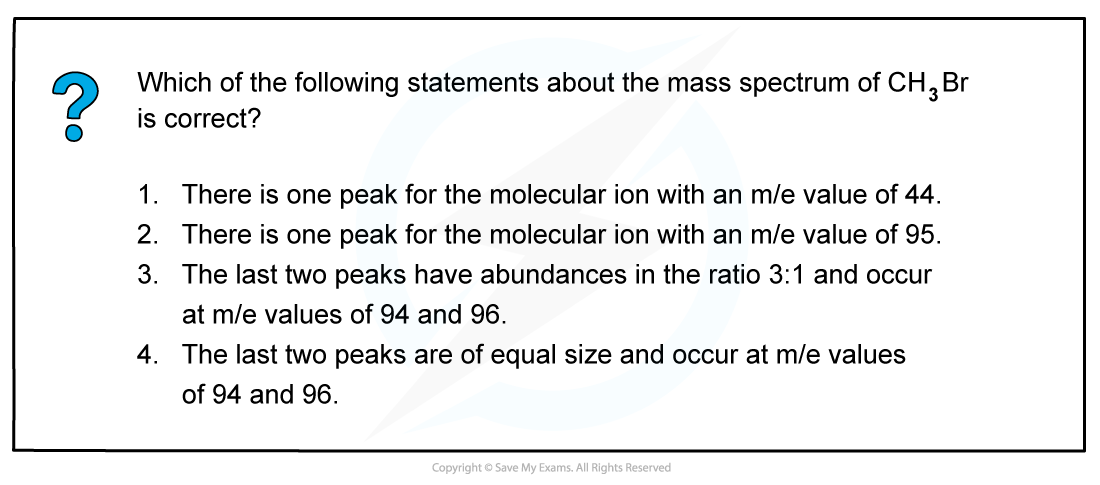The correct answer is 4 as bromomethane (CH3Br) will fragment into 3 peaks

• CH381Br → [CH381Br]+ + e at m/e 96
• CH379Br → [CH379Br]+ + e at m/e 94
• CH3Br → [CH3]+ + •Br at m/e 15

The last two peaks (which correspond to the molecular ion peak) therefore are equal in size and occur at m/e values of 94 and 96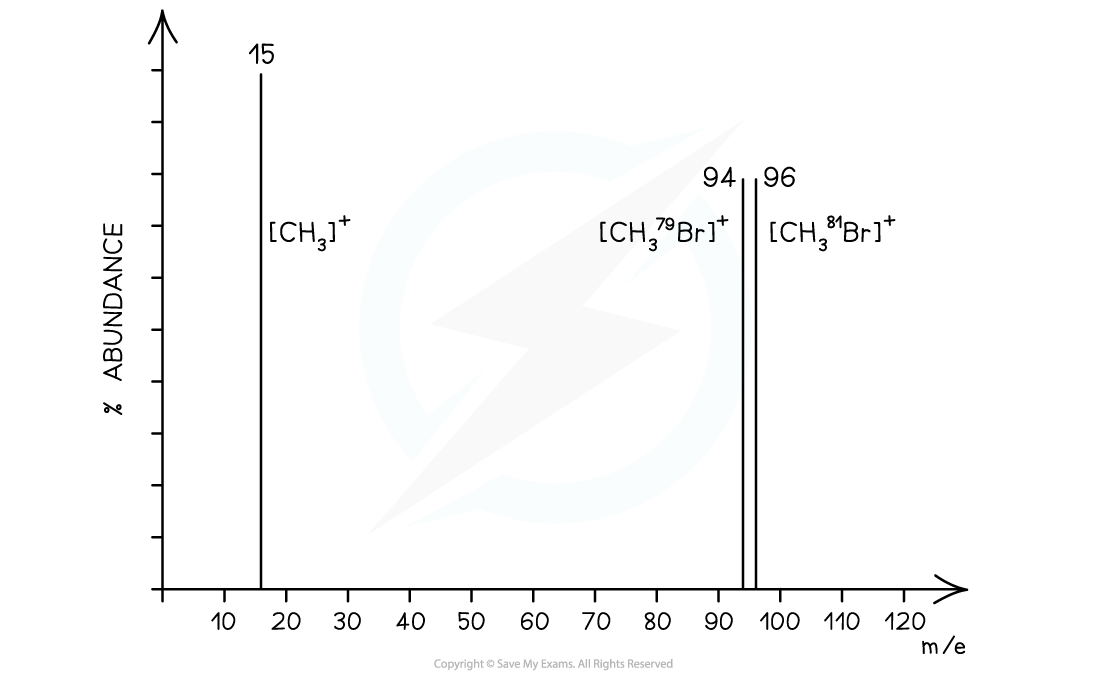#### Worked example: Alcohol fragmentation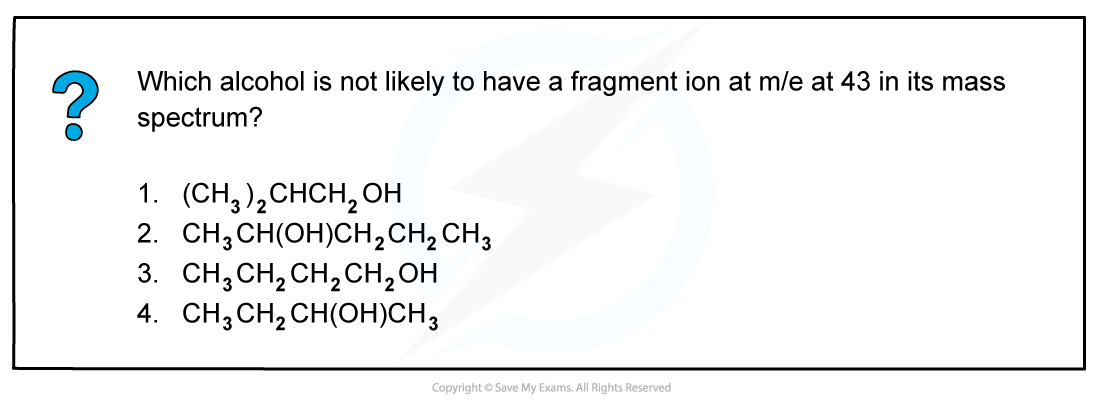The correct answer is 4 because a line at m/e = 43 corresponds to an ion with a mass of 43 for example:

• [CH3CH2CH2]+
• [(CH3)2CH]+

2-butanol is not likely to have a fragment at m/e = 43 as it does not have either of these fragments in its structure.